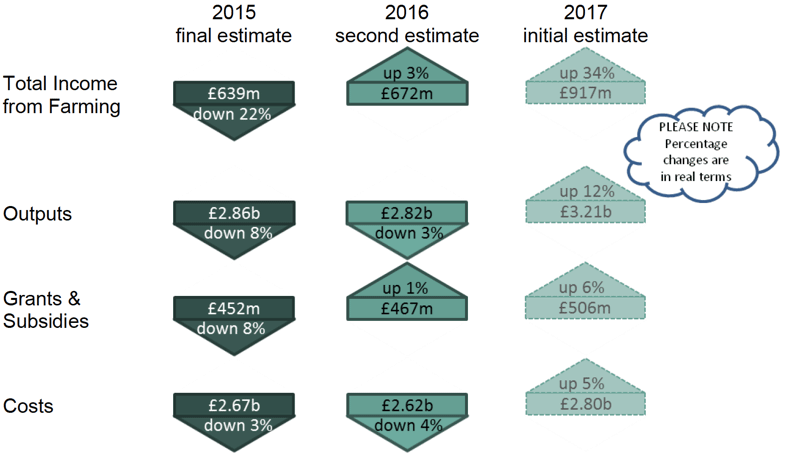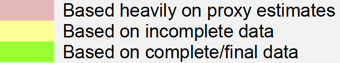# Total income from farming: estimates for Scotland 2015-2017

The official measure of the net income gained by the agriculture industry in Scotland.

### 1. Main Findings

The following summary gives estimates of Total Income from Farming for the latest three years, together with their constituent parts, and the percentage changes after accounting for inflation.Big changes in 2016 Big changes estimated in 2017
Milk down down £53m Milk up £117m
Potatoes up £35m Barley up £67m
Cost of fertilisers down £34m Payments & subsidies up £39m
Sheep up £24m Cost of labour up £34m

Why are the results referred to as "estimates"?

This publication contains three sets of estimates of Total Income from Farming ( TIFF)

final estimates for the calendar year 2015

second estimates for the calendar year 2016

initial estimates for the calendar year 2017.

Many of the data used in calculating income from farming for 2017 will only become available during 2018, and some not until 2019. In particular, the final results of the Farm Business Survey 2017-18, to be used within many of the 2017 costs estimates, are not available until 2019.

This means that the 2017 TIFF estimates published here contain a large number of forecasts, often based on projecting past trends. In January 2019 we will publish updated "second estimates" for 2017, which may differ substantially from those published here. Final estimates for 2017 will then be published in January 2020.

The tables in this publication give a breakdown of the constituent elements of TIFF. In Table 1 we have attempted to illustrate the degree of certainty in the estimates of each element by means of colour coding.Why do initial estimates of TIFF often change so much when they are updated?

TIFF is calculated as income minus costs. Income and costs are similarly-sized large figures, giving a relatively small difference as the value of TIFF. This means that a small percentage update in estimates for income or costs may automatically lead to a large percentage revision in TIFF.

For example, if income = 100 and costs = 96, then TIFF = 4. If we then update the estimate for income upwards by just one per cent, and for costs down by just one per cent, income = 101 and costs = 95, so TIFF = 6, a 50 per cent increase in the value of TIFF.

So reasonable small updates in the estimates of income and costs can lead to what may seem an unreasonable 50 per cent change in TIFF. See also note 4.4.

What does "in real terms" mean?

It shows, for previous years, the value of the income or costs expressed in today's prices. This is because, for example, income of £10,000 in 1990 could have paid for something that nowadays costs £20,000, so we say the 1990 income was worth £20,000 in today's prices.

To do this we simply multiply our data on previous years' figures by the amount prices have gone up since then. In our example prices have doubled, so we multiply the 1990 income by two to see what it would be worth in today's prices. For these multipliers we now use the GDP deflator, from the Office for National Statistics ( ONS)  .